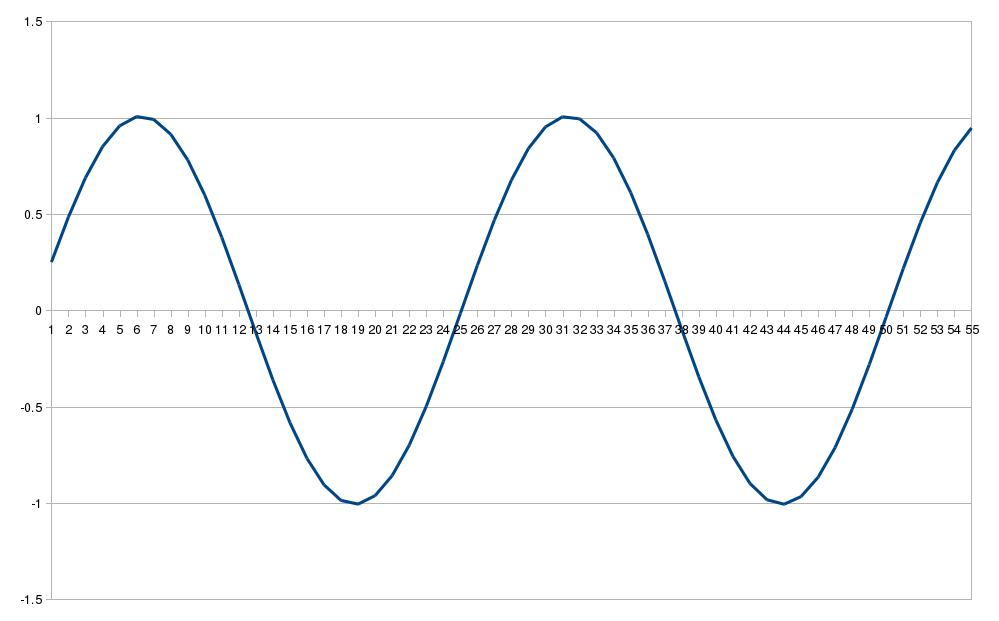5th April 2016

# Performance Comparison C vs. Lua vs. LuaJIT vs. Java

Ico Doornekamp on 20-Dec-2011 asked why a C version of a Lua program ran more slowly than the Lua program. The mentioned discrepancy cannot be reproduced, neither on an AMD FX-8120, nor an Intel i5-4250U processor. Generally a C version program is expected to be faster than a Lua program.

Here is the Lua program called `lua_perf.lua`:

``````local N = 4000
local S = 1000

local t = {}

for i = 0, N do
t[i] = {
a = 0,
b = 1,
f = i * 0.25
}
end

for j = 0, S-1 do
for i = 0, N-1 do
t[i].a = t[i].a + t[i].b * t[i].f
t[i].b = t[i].b - t[i].a * t[i].f
end
print(string.format("%.6f", t.a))
end
``````

It computes values for a circle.Mathematics are in The perfect (sine) wave, or Numerical Solutions of Differential Equations (dead link).

The same program in C called `lua_perf.c`:

``````#include <stdio.h>

#define N 4000
#define S 1000

struct t {
double a, b, f;
};

int main (int argc, char **argv) {
int i, j;
struct t t[N];

for(i=0; i<N; i++) {
t[i].a = 0;
t[i].b = 1;
t[i].f = i * 0.25;
};

for(j=0; j<S; j++) {
for(i=0; i<N; i++) {
t[i].a += t[i].b * t[i].f;
t[i].b -= t[i].a * t[i].f;
}
printf("%.6f\n", t.a);
}

return 0;
}
``````

Same program in Java called `lua_perf.java`:

``````class lua_perf {
public double a, b, f;

static final int N=4000;
static final int S=1000;

public static void main (String[] argv) {
int i, j;
lua_perf[] t = new lua_perf[N];

for(i=0; i<N; i++) {
t[i] = new lua_perf();
t[i].a = 0;
t[i].b = 1;
t[i].f = i * 0.25;
};

for(j=0; j<S; j++) {
for(i=0; i<N; i++) {
t[i].a += t[i].b * t[i].f;
t[i].b -= t[i].a * t[i].f;
}
System.out.println(t.a);
}
}
}
``````

Compile for your machine:

``````cc -Wall -march=native -O3 lua_perf.c -o lua_perf
javac lua_perf.java
``````

Then run the programs multiple times and record the best value.

``````time lua lua_perf.lua > /dev/null

real    0m1.027s
user    0m1.023s
sys     0m0.000s

time luajit lua_perf.lua > /dev/null

real    0m0.042s
user    0m0.040s
sys     0m0.000s

time ./lua_perf > /dev/null

real    0m0.014s
user    0m0.013s
sys     0m0.000s

time java lua_perf > /dev/null

real    0m0.108s
user    0m0.160s
sys     0m0.013s
``````

The result is pretty much as expected: The C program runs three times faster than the LuaJIT program. The LuaJIT program runs almost 25-times faster than the ordinary Lua program.

The Java program needs almost three times as long as LuaJIT. This was totally unexpected. Even when avoiding all the `new` statements in the for-loop, run-time is way higher than LuaJIT. What brings Java back in range to LuaJIT is if one subtracts the Java startup-time. Java startup-time was measured with a program called `lua_perf_empty.java`:

``````class lua_perf_empty {
public static void main (String[] argv) {
System.out.println("Hello, world.");
}
}
``````

This simple program needs 0m0.067s, i.e., startup-time dominates.

``````time java lua_perf_empty > /dev/null

real    0m0.067s
user    0m0.067s
sys     0m0.007s
``````

Startup-time for Lua and LuaJIT is 0m0.002s, i.e., negligible.

C is gcc 5.3.0, Lua is 5.3.2, LuaJIT is 2.0.4, Java is openjdk full version "1.8.0_74-b02".

I also checked all output files for C, Lua, and LuaJIT, i.e., not redirecting to `/dev/null`: All files were identical.

These findings are in line with results given in Julia Benchmarks:Similar results from the LuaJIT website:Comment from Gert Vierman, 23-Apr-2016: Hi, I am the original poster of the message on the Lua list here. The issue was real and reproducible.

“it seems that the code caused a lot of calculations resulting in denormal numbers, which tend to be handled much slower on some hardware . My solution (workaround?) was to enable SSE and add the -ffast-math flag to gcc to tell the compiler I don’t really care about very precise answers.

I’m not sure how denormals affect luajit, but it seems that in this case this is no problem for the luajit implementation.

Comment from Sennie Son, 11-Jul-2019: You are not using the FFI in LuaJITMike Pall has a nice article on his page explaining why using FFI primitives are much faster and memory effective (they are statically typed and fixed size after initialization and thus are way better at being optimized by the JIT) here: https://luajit.org/ext_ffi.html

Resulting code:

``````local ffi = require(“ffi”)
ffi.cdef[[
typedef struct { double a, b, f; } table_elem;
]]

local N = 4000
local S = 1000
local t = ffi.new(“table_elem[?]”, N)

for i = 0, N-1 do
t[i].a = 0.0
t[i].b = 1.0
t[i].f = i * 0.25
end

for j = 0, S-1 do
for i = 0, N-1 do
t[i].a = t[i].a + t[i].b * t[i].f
t[i].b = t[i].b – t[i].a * t[i].f
end
print(string.format(“%.6f”, t.a))
end
``````

Which for me creates a ~4.7x speedup overall.